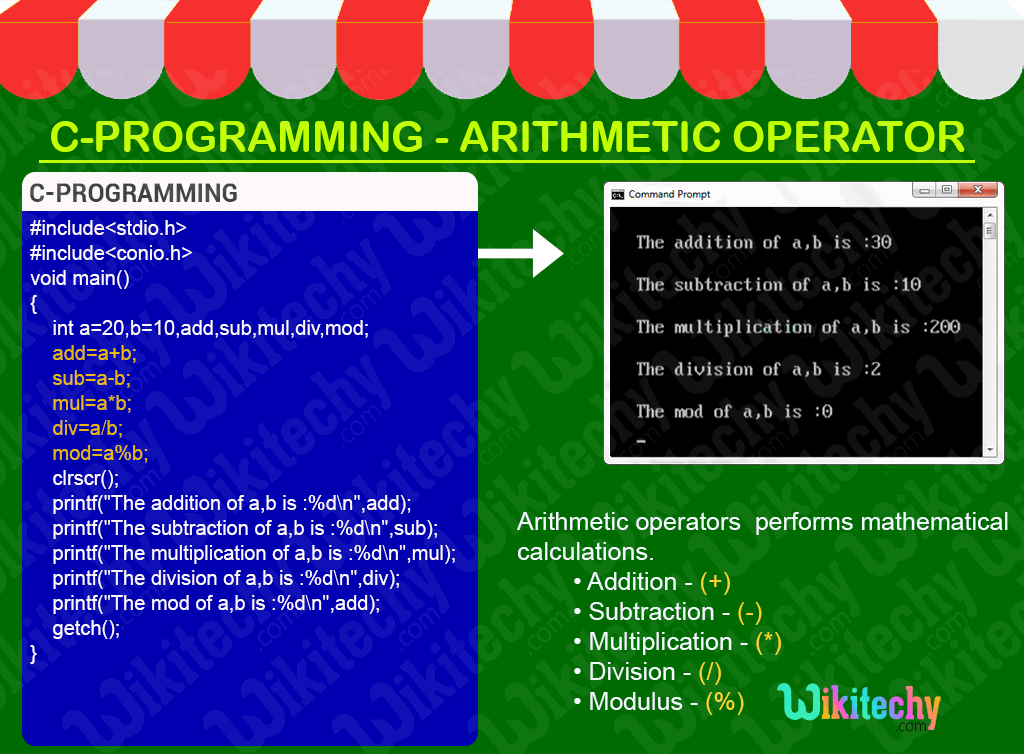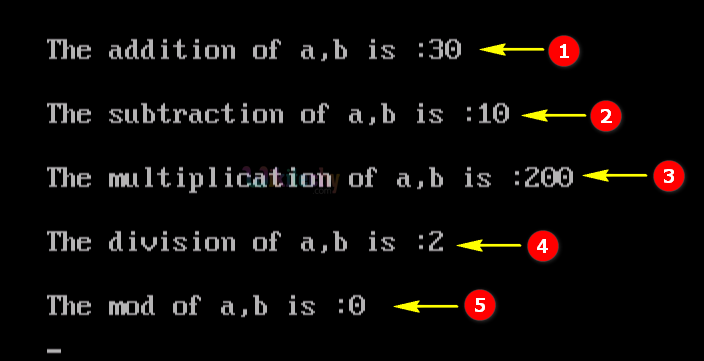# C - Arithmetic OperatorsLearn C - C tutorial - C arithmetic operators - C examples - C programs

## C Arithmetic operators - Definition and Usage

• In C-Programming the Arithmetic operators is used to perform mathematical calculation such as
• Subtraction(-),
• Multiplication(*),
• Division(/) and
• Modulus(%).## C Code - Explanation :1. Here we declare the variable add, sub, mul, div & mod. And assigning the value of the variable a=20 and b=10.
2. In this statement we perform an arithmetic operation for the variable “a” and “b” and the output value will be stored in the variable “add”.
3. In this statement we perform a subtraction operation for the variable “a” and “b” and the output value will be stored in the variable “sub”.
4. In this statement we perform a multiplication operation for the variable “a” and “b” and the output value will be stored in the variable “mul” .
5. In this statement we perform a division operation for the variable “a” and “b” and the output value will be stored in the variable “div” .
6. In this statement we perform a mod operation for the variable “a” and “b” and the output value will be stored in the variable “mod” .
7. Here “print statement” is used for printing the value of the variable “add” in the output console window, same way it displays the output for the sub, mul, div & mod operators.

## Sample Output - Programming Examples1. Here the arithmetic operation for the variable “a” (value=20) and “b” (value=10) has been shown with its output result as “30”.
2. Here the subtraction operation for the variable “a” (value=20) and “b” (value=10) has been shown with its output result as “10”.
3. Here the multiplication operation for the variable “a” (value=20) and “b” (value=10) has been shown with its output result as “200”.
4. Here the division operation for the variable “a” (value=20) and “b” (value=10) has been shown with its output result as “2”.
5. Here the mod operation for the variable “a” (value=20) and “b” (value=10) has been shown with its output result as “0”.